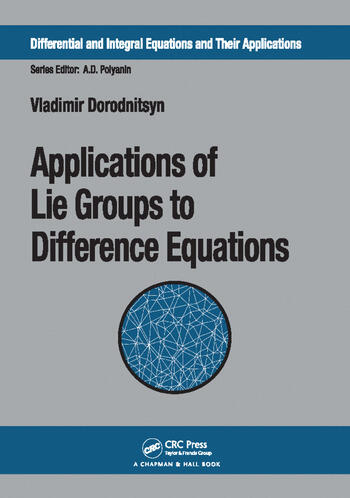Applications of Lie Groups to Difference Equations

1st Edition

Chapman and Hall/CRC
Published June 16, 2017
Reference - 344 Pages - 31 B/W Illustrations
ISBN 9781138118232 - CAT# K35588
Series: Differential and Integral Equations and Their Applications

For Instructors Request Inspection Copy

was \$82.95

USD\$66.36

SAVE ~\$16.59

FREE Standard Shipping!

Preview

Summary

Intended for researchers, numerical analysts, and graduate students in various fields of applied mathematics, physics, mechanics, and engineering sciences, Applications of Lie Groups to Difference Equations is the first book to provide a systematic construction of invariant difference schemes for nonlinear differential equations. A guide to methods and results in a new area of application of Lie groups to difference equations, difference meshes (lattices), and difference functionals, this book focuses on the preservation of complete symmetry of original differential equations in numerical schemes. This symmetry preservation results in symmetry reduction of the difference model along with that of the original partial differential equations and in order reduction for ordinary difference equations.

A substantial part of the book is concerned with conservation laws and first integrals for difference models. The variational approach and Noether type theorems for difference equations are presented in the framework of the Lagrangian and Hamiltonian formalism for difference equations.

In addition, the book develops difference mesh geometry based on a symmetry group, because different symmetries are shown to require different geometric mesh structures. The method of finite-difference invariants provides the mesh generating equation, any special case of which guarantees the mesh invariance. A number of examples of invariant meshes is presented. In particular, and with numerous applications in numerics for continuous media, that most evolution PDEs need to be approximated on moving meshes.

Based on the developed method of finite-difference invariants, the practical sections of the book present dozens of examples of invariant schemes and meshes for physics and mechanics. In particular, there are new examples of invariant schemes for second-order ODEs, for the linear and nonlinear heat equation with a source, and for well-known equations including Burgers equation, the KdV equation, and the Schrödinger equation.

Instructors

We provide complimentary e-inspection copies of primary textbooks to instructors considering our books for course adoption.